Solving Equations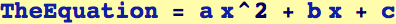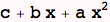Note the use of Equal (==) rather than Set (=) in the following; using "=" will produce an error message.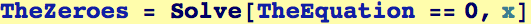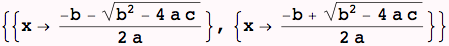Note that the roots are given as Rules. Now we ask Mathematica to verify that the solutions it found are indeed roots to the specified equation. Here is a prototypical example of using Replace (/.) to accomplish this: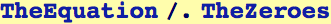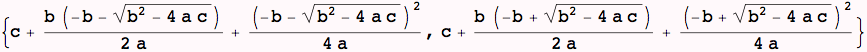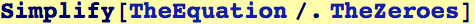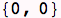More examples of using Solve: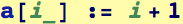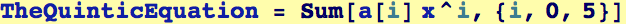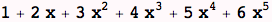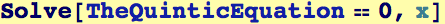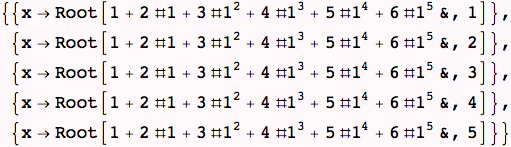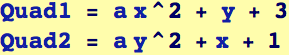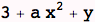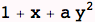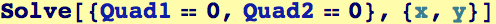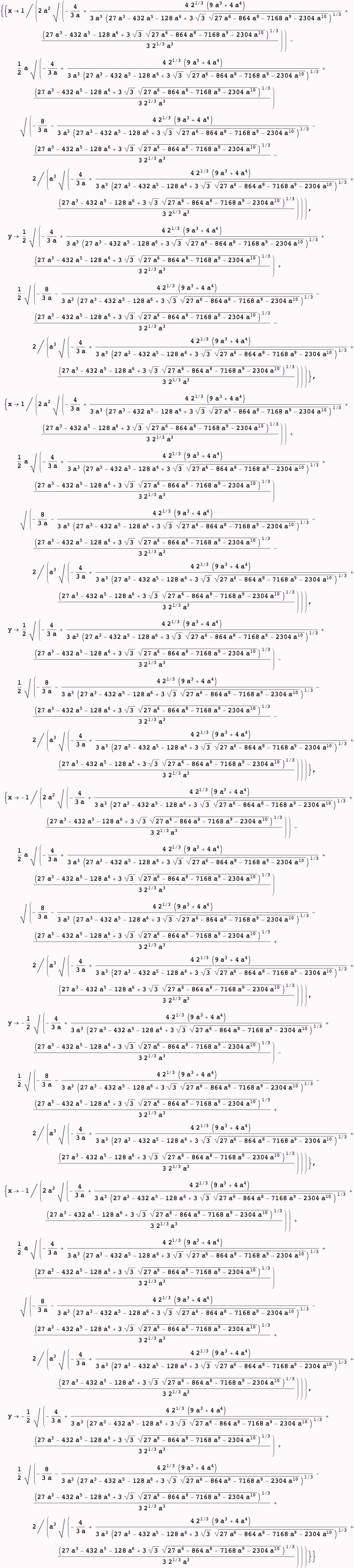Created by Wolfram Mathematica 6.0  (28 August 2007)